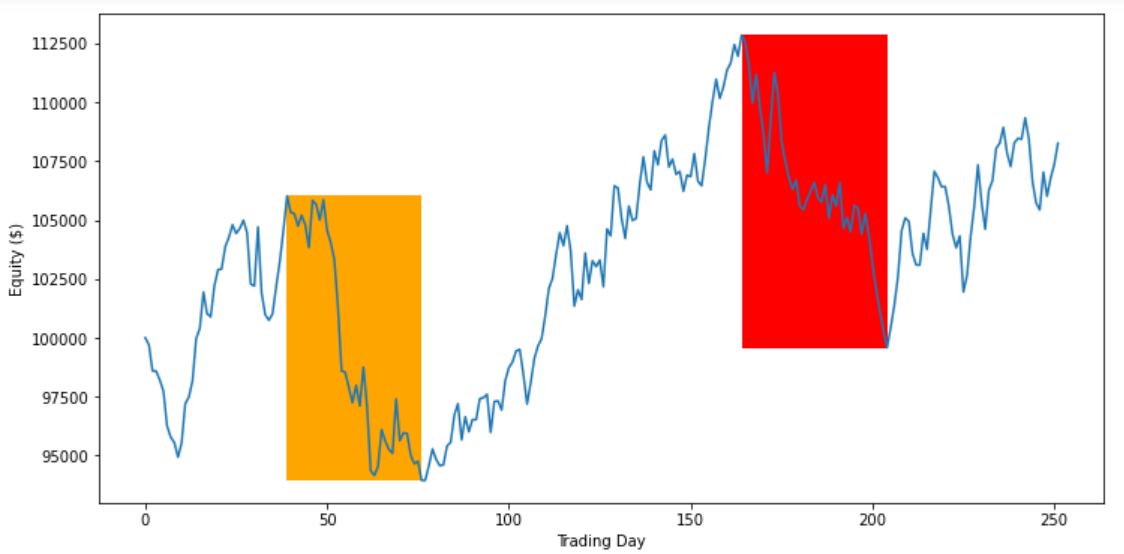# Optimization

## Objectives

### Introduction

An optimization objective is the performance metric that's used to compare the backtest performance of different parameter values. The optimizer currently supports the compound annual growth rate (CAGR), drawdown, Sharpe ratio, and Probabilistic Sharpe ratio (PSR) as optimization objectives. When the optimization job finishes, the results page displays the value of the objective with respect to the parameter values.

### CAGR

CAGR is the yearly return that would be required to generate the return over the backtest period. CAGR is calculated as

$$(\frac{e}{s})^{\frac{1}{y}}-1$$

where $s$ is starting equity, $e$ is ending equity, and $y$ is the number of years in the backtest period. The benefit of using CAGR as the objective is that it maximizes the return of your algorithm over the entire backtest. The drawback of using CAGR is that it may cause your algorithm to have more volatile returns, which increases the difficulty of keeping your algorithm deployed in live mode.

### Drawdown

Drawdown is the largest peak to trough decline in your algorithm's equity curve. Drawdown is calculated as

$$1- \frac{v^{t\ge s}_{min}}{v^s_{max}}$$

where $v^s_{max}$ is the maximum equity value up to time $s$ and $v^{t\ge s}_{min}$ is the minimum equity value at time $t$ where $t\ge s$. The following image illustrates how the max drawdown is calculated:During the first highlighted period in the preceding image, the equity curve dropped from 106,027 to 93,949 (11.4%). During the second highlighted period, the equity curve dropped from 112,848 to 99,576 (11.8%). Since 11.8% > 11.4%, the max drawdown of the equity curve is 11.8%.

The benefit of using drawdown as the objective is that it's psychologically easier to keep an algorithm deployed in live mode if the algorithm doesn't experience large drawdowns. The drawback of using drawdown is that it may limit the potential returns of your algorithm.

### Sharpe

The Sharpe ratio measures the excess returns relative to a benchmark, divided by the standard deviation of those returns. The Sharpe ratio is calculated as

$$\frac{E[R_p - R_b]}{\sigma_p}$$

where $R_p$ is the returns of your portfolio, $R_b$ is the returns of the benchmark, and $\sigma_p$ is the standard deviation of your algorithm's excess returns. By default, Lean uses a 0% risk-free rate, so $R_b=0$. The benefit of using the Sharpe ratio as the objective is that it maximizes returns while minimizing the return volatility. It's usually psychologically easier to keep a live algorithm deployed if it has minimal swings in equity than if it has large swings in equity. The drawback of using the Sharpe ratio is that it may limit your potential returns in favor of a less volatile equity curve.

### PSR

The PSR is the probability that the estimated Sharpe ratio of your algorithm is greater than the Sharpe ratio of the benchmark. PSR is calculated as

$P\left(\hat{SR} > SR^{\ast}\right) = CDF\left(\frac{(\hat{SR} - SR^{\ast})\sqrt{n-1}}{\sqrt{1 - \hat{\gamma}_{3}\hat{SR} + \frac{\hat{\gamma}_{4}-1}{4}\hat{SR}^{2}}}\right)$

where $SR^{\ast}$ is the Sharpe ratio of the benchmark, $\hat{SR}$ is the Sharpe ratio of your algorithm, $n$ is the number of trading days, $\hat{\gamma}_{3}$ is the skewness of your algorithm's returns, $\hat{\gamma}_{4}$ is the kurtosis of your algorithm's returns, and $CDF$ is the normal cumulative distribution function. The benefit of using the PSR as the objective is that it maximizes the probability of your algorithm's Sharpe ratio outperforming the benchmark Sharpe ratio. The drawback of using the PSR is that, like the Sharpe ratio objective, optimizing the PSR may limit your potential returns in favor of a less volatile equity curve.

### Constraints

Constraints filter out backtests from your optimization results that do not conform to your desired range of statistical results. Constraints consist of a target, operator, and a numerical value. For instance, you can add a constraint that the optimization backtests must have a Sharpe ratio >= 1 to be included in the optimization results. Constraints are optional, but you can use them to incorporate multiple objective functions into a single optimization job.

You can also see our Videos. You can also get in touch with us via Discord.